2 November 2019

# xsections and layers

### How to create waveguide definitions for layout

##### xsections and layers

Many, if not most, waveguides and metal lines in a technology consist of more than one mask layer. By added one or more layers to a xsection (cross section) object it becomes possible to draw those layers at the same time in the context of the xsection. To make this all work we need to define a xsection, one or more layers, and add layers to the xsection. Nazca allows you to do it all at once, as it will automatically create xsections and layers it hasn’t encountered earlier.

By default, a layer in a xsection will have a certain width. However,  it is possible to grow the width of layers when adding them to a xsection. An example of this concept is a waveguide of a specific width and a trench layer that is a wider version of the waveguide. Below is an example of a xsection having 3 mask layers. The growx keyword is used to describe the symmetrical widening of the guide with respect the default width, where the value represents the growth per side.

The layer has an “accuracy” attribute that describes its resolution in the mask layout in um. A default accuracy will be used if the call omits a value.

```# example created by Bright Photonics

import nazca as nd

# create layers in a xsection

nd.strt(length=10, xs='XS1').put(0)

nd.export_gds()```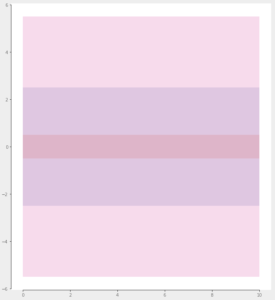Instead of having Nazca creating unknown xsections and/or layers as in the example above, it is better practice to explicitly define them as in the code below. The next example also shows how to create an interconnect from the newly defined xsection and how to draw mask elements with the interconnect.

```# example created by Bright Photonics

import nazca as nd

# create a xsection

# create layers

# combine layers in a xsection

# create an interconnect object

# create some layout
ic1.strt(length=10).put(0)
ic1.bend(angle=10).put()
ic1.taper(length=15, width2=4.0).put()

nd.export_gds()```

The next example will have the same output as the previous, but new is that we assign width and radius attributes to the xsection object. The object is fetched in variable xs1 when creating the new xsection. The interconnect definition will reuse the data available in xs1. To overrule the xsection settings in an interconnect, the “width” and “radius” values can still be provided by keyword assignment as in the previous example.

```# example created by Bright Photonics

import nazca as nd

# create a xsection
xs1.width = 3.0

# create layers

# combine layers in a xsection

# create an interconnect object
ic1 = nd.interconnects.Interconnect(xs='XS1')

# create some layout
ic1.strt(length=10).put(0)
ic1.bend(angle=10).put()
ic1.taper(length=15, width2=4.0).put()

nd.export_gds()```

To have more control in defining the width and/or growth of the waveguide, instead of “growx” the “leftedge” and “rightedge” keywords are available. Each edge is described as y = a*width + b, with “width” the default width. the constants a and b are provided as tuple (a, b). The example below shows how this works reproducing the same output as the previous two examples. Note that interchanging the right and left edge result in the same polygon shape but a reversed order of the polygon points.

```# example created by Bright Photonics

import nazca as nd

# create a xsection
xs1.width = 3.0

# create layers

# combine layers in a xsection
# from 0.5*width to -0.5*width:
# from 0.5*width+2.0 to -0.5*width-2.0:
nd.add_layer2xsection(xsection='XS1', layer='L2', leftedge=(0.5, 2), rightedge=(-0.5, -2))
# from 0.5*width+5.0 to -0.5*width-5.0
nd.add_layer2xsection(xsection='XS1', layer='L3', leftedge=(0.5, 5), rightedge=(-0.5, -5))

# create an interconnect object
ic1 = nd.interconnects.Interconnect(xs='XS1')

# create some layout
ic1.strt(length=10).put(0)
ic1.bend(angle=10).put()
ic1.taper(length=15, width2=4.0).put()

nd.export_gds()```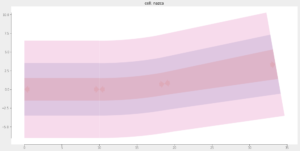Having the “leftedge” and “rightedge” option we can also define asymmetric guides, e.g. for trenching on one side, as shown in the next example.

```# example created by Bright Photonics

import nazca as nd

# create a xsection
xs1.width = 3.0

# create layers

# combine layers in a xsection
nd.add_layer2xsection(xsection='XS1', layer='L2', leftedge=(0.5, 0), rightedge=(1.5, 0))
nd.add_layer2xsection(xsection='XS1', layer='L3', leftedge=(1.0, -4), rightedge=(-1.0, -4))

# create an interconnect object
ic1 = nd.interconnects.Interconnect(xs='XS1')

# create some layout
ic1.strt(length=10).put(0)
ic1.bend(angle=10).put()
ic1.taper(length=15, width2=4.0).put()

nd.export_gds()```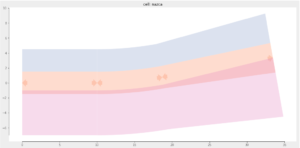The next example shows how the layer in xsection definition is exploited to draw an RF-line structure. A layer can be resused in a xsection to define multiple waveguide structures.

On top of that the example shows that a layer in a xsection has a “polyline” property (as in Nazca-0.5.6 for strt, bend and taper). If polyline is set to True (default=False), the content of the layer is exported to a gds polyline (gds path) instead of a polygon. In the figure the polyline is visible at the bottom, in layer ‘L3’. Hence, it is possible to define polygon waveguide layers and polylines in a single xsection. Note that a polyline by definition has a fixed width, so tapers will be transformed in fixed width lines.

```# example created by Bright Photonics

import nazca as nd

# create a xsection
xs1.width = 3.0

# create layers

# combine layers in a xsection
nd.add_layer2xsection(xsection='XS1', layer='L1', leftedge=(3.0, 1.0), rightedge=(1.0, 1.0))
nd.add_layer2xsection(xsection='XS1', layer='L1', leftedge=(-1.0, -1.0), rightedge=(-3.0, -1.0))
nd.add_layer2xsection(xsection='XS1', layer='L2', leftedge=(4.0, 1.0), rightedge=(-4.0, -1.0))
nd.add_layer2xsection(xsection='XS1', layer='L3', leftedge=(0.0, -14.0), rightedge=(0.0, -16.0), polyline=True)

# create an interconnect object
ic1 = nd.interconnects.Interconnect(xs='XS1')

# create some layout
ic1.strt(length=10).put(0)
ic1.bend(angle=10).put()
ic1.taper(length=15, width2=4.0).put()

nd.export_gds()```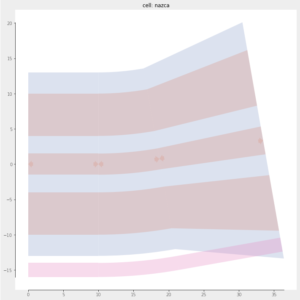All layers in the section taper and curve just fine along the a*width + b definition. If you want to connect to lines having a different edge definition, but the same layers and layer order, it is possible to connect two different interconnect definitions as shown in the code below, utilizing the interconnect “connect” method.

```# example created by Bright Photonics

import nazca as nd

# create two xsections
xs1.width = 3.0
xs2.width = 3.0

# create layers

# combine layers in xsection 'XS1'
nd.add_layer2xsection(xsection='XS1', layer='L1', leftedge=(3.0, 1.0), rightedge=(2.5, 1.0))
nd.add_layer2xsection(xsection='XS1', layer='L1', leftedge=(-1.0, 0.0), rightedge=(-3.0, -1.0))
nd.add_layer2xsection(xsection='XS1', layer='L2', leftedge=(4.0, 1.0), rightedge=(-4.0, -1.0))
nd.add_layer2xsection(xsection='XS1', layer='L3', leftedge=(0.0, -14.0), rightedge=(0.0, -16.0), polyline=True)

# combine layers in xsection 'XS2'
nd.add_layer2xsection(xsection='XS2', layer='L1', leftedge=(0.75, 0.0), rightedge=(-1.0, 0.0))
nd.add_layer2xsection(xsection='XS2', layer='L1', leftedge=(3.0, 0.0), rightedge=(1.0, 0.0))
nd.add_layer2xsection(xsection='XS2', layer='L1', leftedge=(-2.0, -1.0), rightedge=(-2.0, -3.0))
nd.add_layer2xsection(xsection='XS2', layer='L2', leftedge=(4.0, 0.0), rightedge=(-2.0, -4.0))
nd.add_layer2xsection(xsection='XS2', layer='L3', leftedge=(0.0, -14.0), rightedge=(0.0, -16.0), polyline=True)

# create interconnect objects for 'XS1' and 'XS2'
ic1 = nd.interconnects.Interconnect(xs='XS1')
ic2 = nd.interconnects.Interconnect(xs='XS2')

# create some layout
ic1.strt(length=5).put(0)
ic1.bend(angle=20).put()
ic1.taper(length=15, width2=4.0).put()
ic1.strt(length=15, width=4.0).put()
ic1.connect(width1=4.0, length=30, ic=ic2).put() # go from ic1 to ic2 having the same layer order.
ic2.bend(angle=-20).put()
ic2.taper(width2=2.0, length=25).put()
ic2.strt(width=2.0, length=5.0).put()

nd.export_gds()```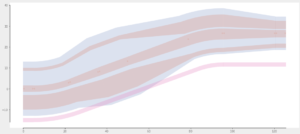## Related Tutorials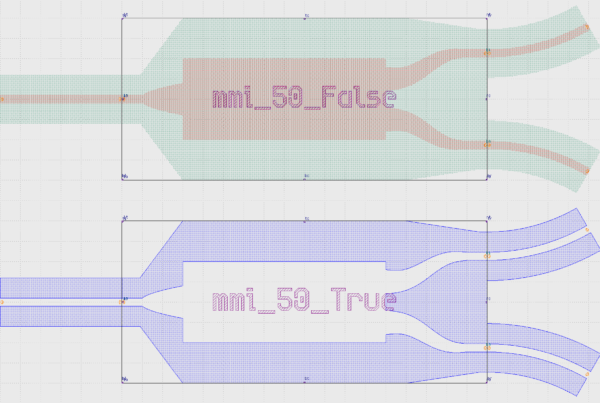In this example we show how to create an inverted MMI with custom interconnects.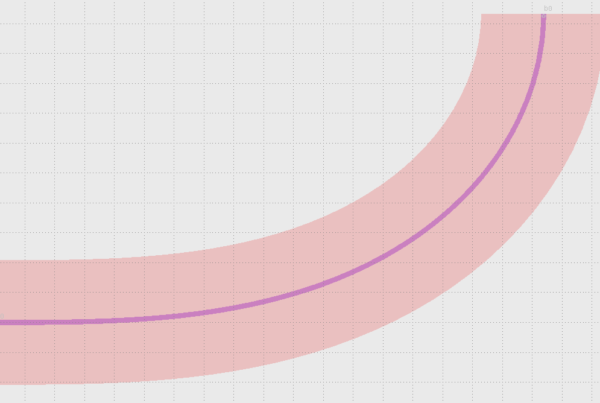In this example we show how to use Euler bends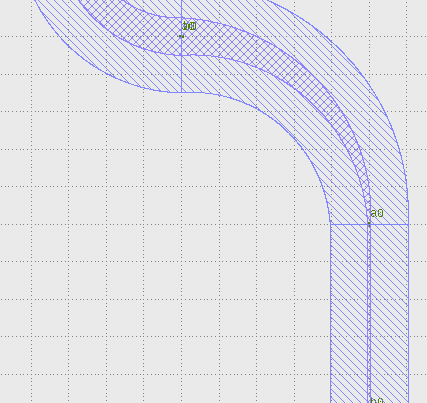In this example we show how to create a free form parametric curve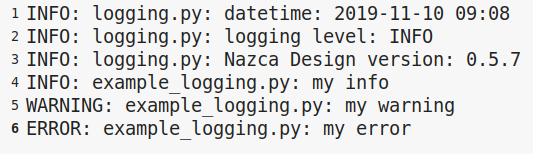In this example we show how to log your layout.